# Visualizing Prime Numbers

Visualization of prime numbers - is there a pattern?

# Introduction

This probably doesn’t fall strictly into the realm of data science, but I thought it would be interesting to look at prime numbers.

# Visualization

I wanted to see where primes occurred in a range of numbers, and a simple way to do that was to create a heatmap, using 0s to represent composites and 1s for primes. To do this, I’ll use seaborn, and I’ll work with numbers that are perfect squares, so that it can be represented in a square heatmap.

# Steps

1. Write a function to check if a number is a prime number
2. Write a function to generate a list of prime numbers up to a limit
3. Write a function to generate a square matrix of numbers in running order, starting from 1
4. Write a function to generate the matrix we’ll use for the heatmap
• The shape of this matrix should be the same as the square matrix we generated earlier
• Our list of prime numbers is like a mask
• Where the number is prime, substitute it with 1, otherwise 0 (for maximum difference in color on the heatmap)
5. Using the matrix generated in the previous step, generate the heatmap

• the value of a square is the sum of the value on the x-axis and y-axis
• dark squares are prime numbers, light squares are composite numbers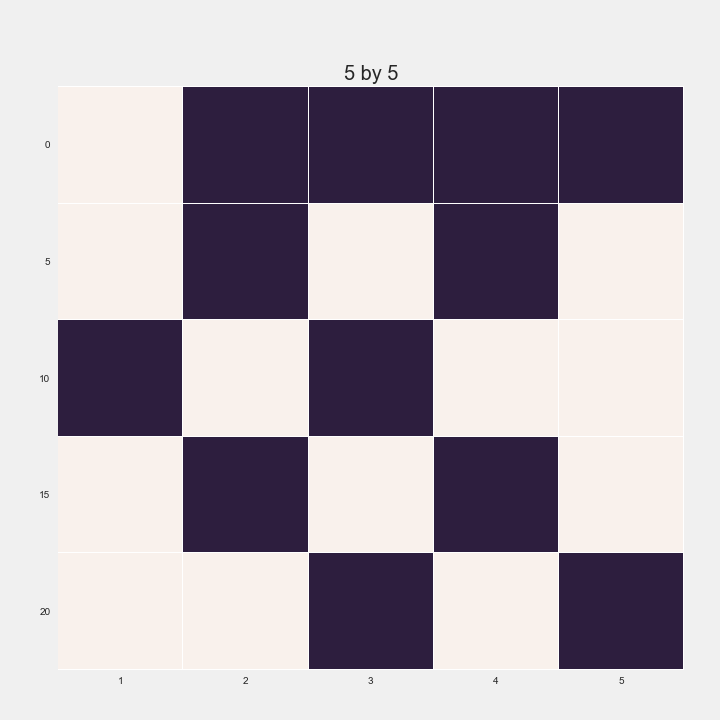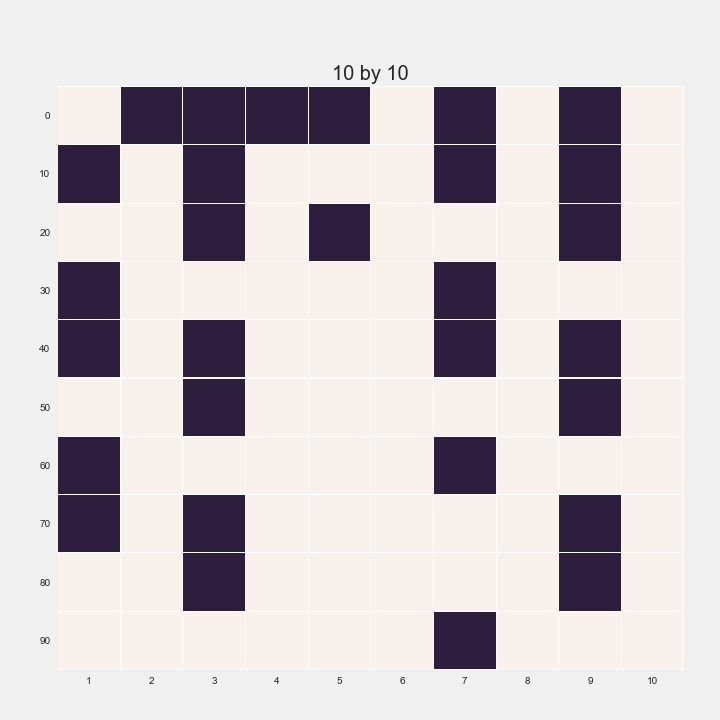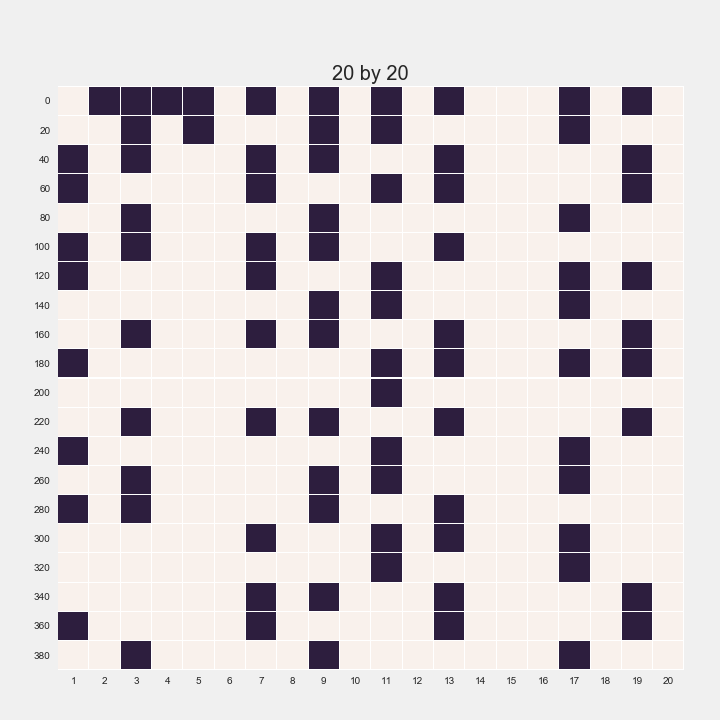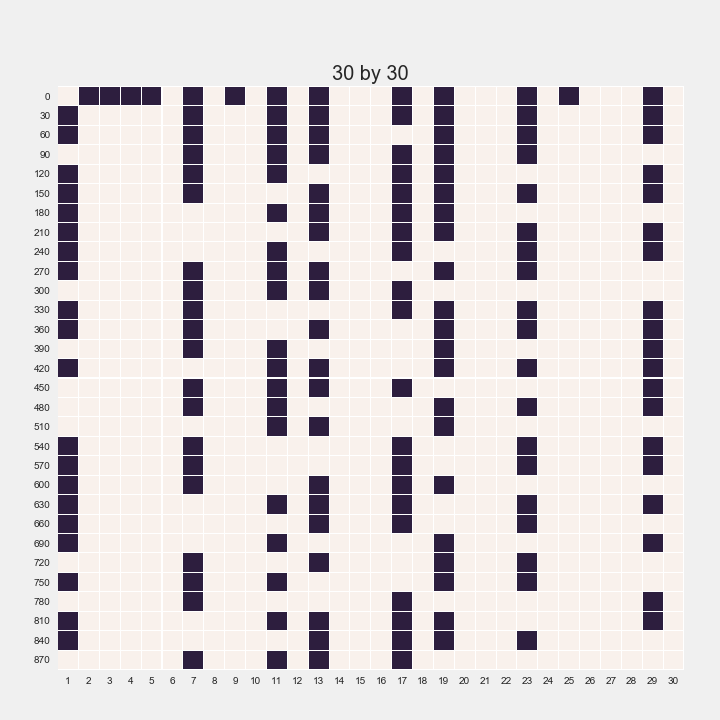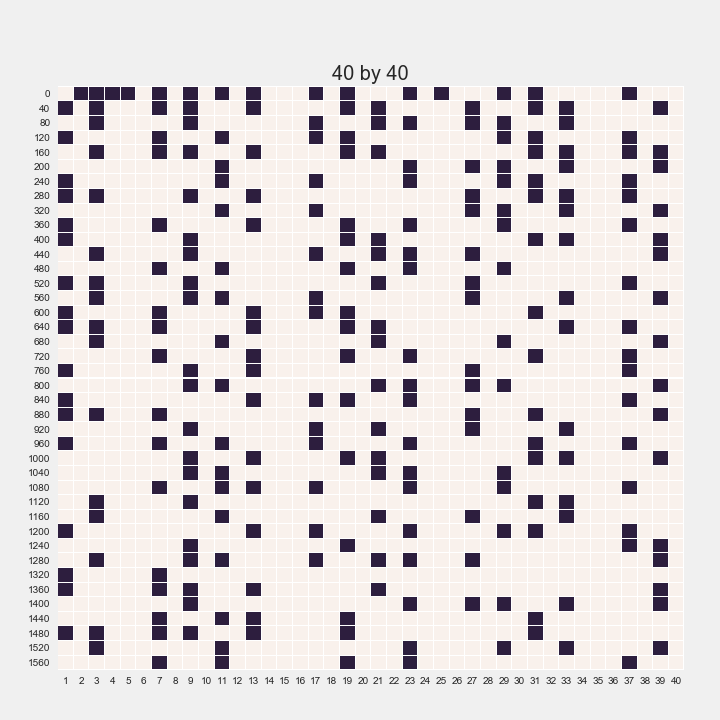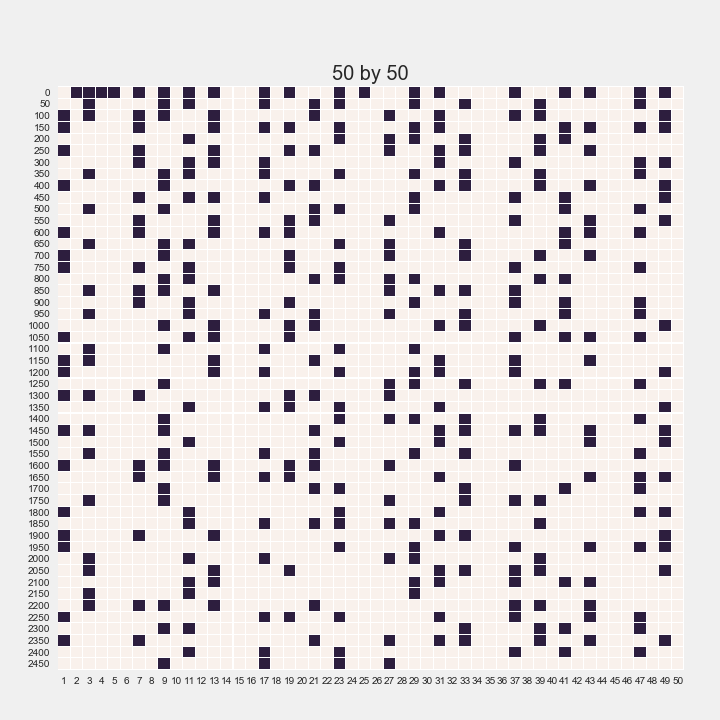# Observations

• at first there seems to be no discernible pattern, it’s just random spots all over the sqaure
• at the higher numbers, there are columns with more primes than others
• there are also columns with no primes at all
• given that the x and y axes are added together to get the value of a square, the x-axis will give us the ending figure of a value
• rows look more random than columns

# Number of primes

How do the number of primes change as N gets bigger?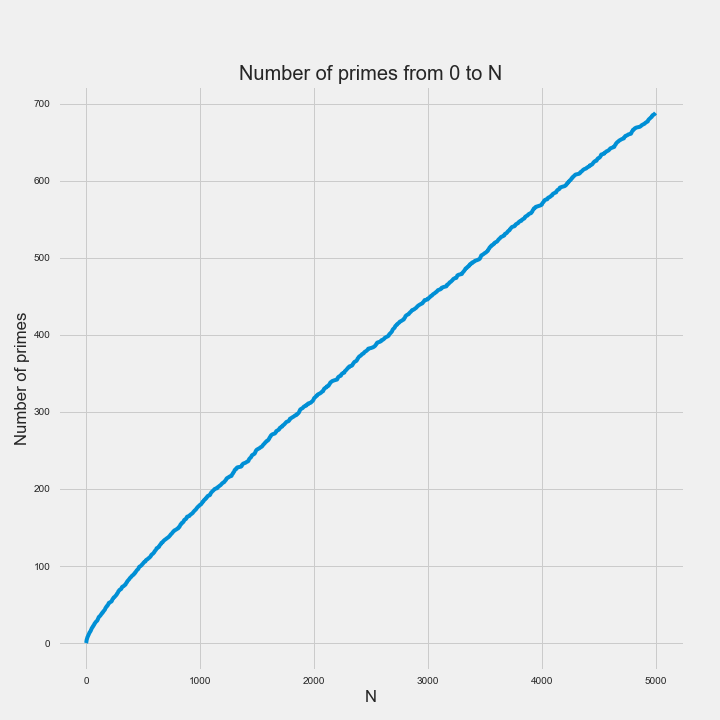• it looks linear on first glance
• but at lower N, the number of primes is increasing at a faster rate than at a higher N
• N is not very big right now (because of runtime constraints), but it looks like as N gets bigger, the number of primes is just going to keep increasing
• note that at a big enough N, it could be totally possible that the number of primes starts to plateau (but I think they haven’t found that N yet)

Thank you for reading and I hope this was interesting! You can find my notebook on GitHub. Let me know if you have any comments or suggestions!

Tags:

Updated: